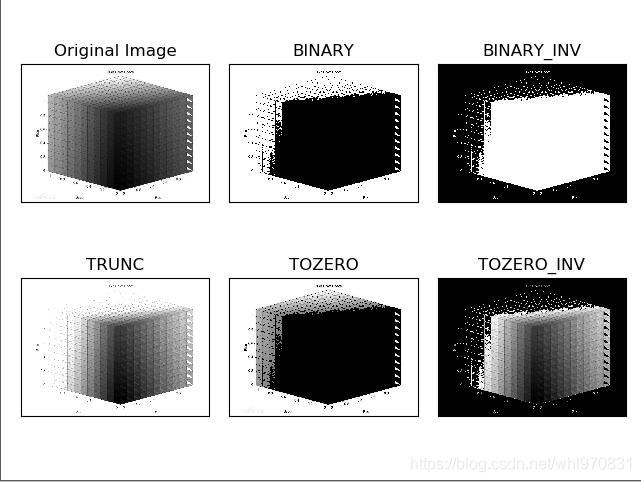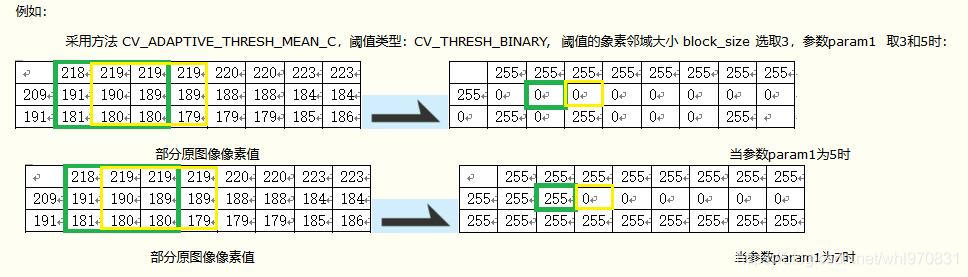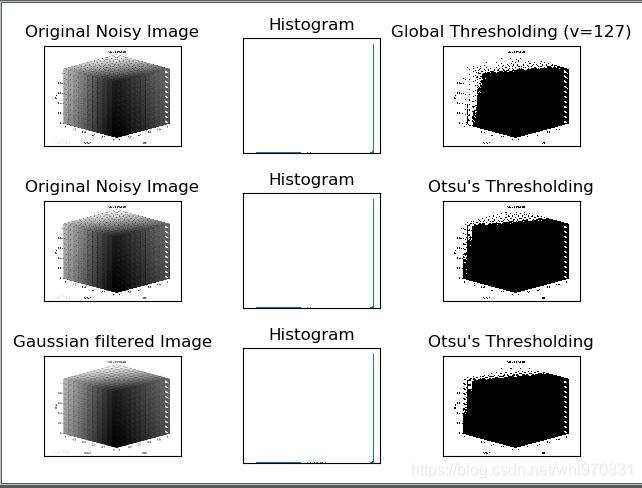# OpenCV学习心得——python版——图像二值化（图像阈值）——全局阈值与自适应阈值

OpenCV学习心得——python版——图像二值化（图像阈值）——全局阈值与自适应阈值
FOR THE SIGMA
FOR THE GTINDER
FOR THE ROBOMASTER

## 简介：

opencv-python2.xx.xx以上（我用的最新的，但是不一定要用最新的，3.4左右版本就行了）
matplotlib（图表工具）
numpy
pillow

## 内容：

• 所谓图像的二值化 ，就是将图像上的像素点的灰度值设置为0或255，也就是将整个图像呈现出明显的只有黑和白的视觉效果。一幅图像包括目标物体、背景还有噪声，要想从多值的数字图像中直接提取出目标物体。
• 全局阈值就是一幅图像包括目标物体、背景还有噪声，要想从多值的数字图像中直接提取出目标物体；常用的方法就是设定一个阈值T，用T将图像的数据分成两部分：大于T的像素群和小于T的像素群。这是研究灰度变换的最特殊的方法，称为图像的二值化（Binarization）
• 局部阈值就是当同一幅图像上的不同部分的具有不同亮度时。这种情况下我们需要采用自适应阈值。此时的阈值是根据图像上的每一个小区域计算与其对应的阈值。因此在同一幅图像上的不同区域采用的是不同的阈值，从而使我们能在亮度不同的情况下得到更好的结果。

#### 简单阈值处理（全局阈值）：

Python-OpenCV中提供了阈值（threshold）函数：

• cv2.threshold（）
• 函数：
• 第一个参数 src 指原图像，原图像应该是灰度图。
• 第二个参数 x 指用来对像素值进行分类的阈值。
• 第三个参数 y 指当像素值高于（有时是小于）阈值时应该被赋予的新的像素值
• 第四个参数 Methods 指不同的不同的阈值方法，这些方法包括：
• cv2.THRESH_BINARY
• cv2.THRESH_BINARY_INV
• cv2.THRESH_TRUNC
• cv2.THRESH_TOZERO
• cv2.THRESH_TOZERO_INV
``````import cv2
from matplotlib import pyplot as plt #用matplotible显示图标

ret,thresh1 = cv2.threshold(img,127,255,cv2.THRESH_BINARY)
ret,thresh2 = cv2.threshold(img,127,255,cv2.THRESH_BINARY_INV)
ret,thresh3 = cv2.threshold(img,127,255,cv2.THRESH_TRUNC)
ret,thresh4 = cv2.threshold(img,127,255,cv2.THRESH_TOZERO)
ret,thresh5 = cv2.threshold(img,127,255,cv2.THRESH_TOZERO_INV)

titles = ['Original Image','BINARY','BINARY_INV','TRUNC','TOZERO','TOZERO_INV'] #标题
images = [img, thresh1, thresh2, thresh3, thresh4, thresh5] #对应的图

for i in range(6): #画4次图
plt.subplot(2,3,i+1),plt.imshow(images[i],'gray')
plt.title(titles[i])
plt.xticks([]),plt.yticks([])

plt.show()
``````#### 自适应阈值（局部阈值）：

Python-OpenCV中提供了自适应阈值函数：

• 函数：
• 第一个参数 src 指原图像，原图像应该是灰度图。
• 第二个参数 x 指当像素值高于（有时是小于）阈值时应该被赋予的新的像素值
• 第四个参数 threshold_type 指取阈值类型：必须是下者之一
• CV_THRESH_BINARY
• CV_THRESH_BINARY_INV
• 第五个参数 block_size 指用来计算阈值的象素邻域大小: 3, 5, 7, …
• 第六个参数 param1 指与方法有关的参数。``````import cv2
from matplotlib import pyplot as plt
#详细说明参考上方例子

img = cv2.medianBlur(img,5)

ret,th1 = cv2.threshold(img,127,255,cv2.THRESH_BINARY)

cv2.THRESH_BINARY,11,2)

cv2.THRESH_BINARY,11,2)

titles = ['Original Image', 'Global Thresholding (v = 127)',
images = [img, th1, th2, th3]

for i in range(4):
plt.subplot(2,2,i+1),plt.imshow(images[i],'gray')
plt.title(titles[i])
plt.xticks([]),plt.yticks([])
plt.show()
``````

``````import cv2 as cv

#全局阈值
def threshold_demo(image):
gray = cv.cvtColor(image, cv.COLOR_RGB2GRAY)  #把输入图像灰度化
ret, binary = cv.threshold(gray, 0, 255, cv.THRESH_BINARY | cv.THRESH_TRIANGLE) #直接阈值化是对输入的单通道矩阵逐像素进行阈值分割。

#print("threshold value %s"%ret)
cv.namedWindow("threshold", cv.WINDOW_NORMAL)
cv.imshow("threshold", binary)

#局部阈值
def local_threshold(image):
gray = cv.cvtColor(image, cv.COLOR_RGB2GRAY)  #把输入图像灰度化

cv.namedWindow('input_image', cv.WINDOW_NORMAL) #设置为WINDOW_NORMAL可以任意缩放

cv.imshow('input_image', src) #源图
threshold_demo(src) #全局
local_threshold(src) #局部

cv.waitKey(0)
cv.destroyAllWindows()

``````

## 扩展：

#### Otsu 二值化

• 在使用全局阈值时，我们就是随便给了一个数来做阈值，那我们怎么知道我们选取的这个数的好坏呢？答案就是不停的尝试。如果是一副双峰图像（简单来说双峰图像是指图像直方图中存在两个峰）呢？我们岂不是应该在两个峰之间的峰谷选一个值作为阈值？这就是 Otsu 二值化要做的。简单来说就是对一副双峰图像自动根据其直方图计算出一个阈值。（对于非双峰图像，这种方法得到的结果可能会不理想）。
• 这里用到到的函数还是 cv2.threshold()，但是需要多传入一个参数（flag）：cv2.THRESH_OTSU。
• 这时要把阈值设为 0。然后算法会找到最优阈值，这个最优阈值就是返回值 retVal。如果不使用 Otsu 二值化，返回的retVal 值与设定的阈值相等。
下面的例子中，输入图像是一副带有噪声的图像。第一种方法，设127 为全局阈值。第二种方法，直接使用 Otsu 二值化。第三种方法，先使用一个 5x5 的高斯核除去噪音，然后再使用 Otsu 二值化。
``````import cv2
from matplotlib import pyplot as plt

# 设127 为全局阈值
ret1,th1 = cv2.threshold(img,127,255,cv2.THRESH_BINARY)

# 直接使用 Otsu 二值化
ret2,th2 = cv2.threshold(img,0,255,cv2.THRESH_BINARY+cv2.THRESH_OTSU)

# 先使用一个 5x5 的高斯核除去噪音，然后再使用 Otsu 二值化
blur = cv2.GaussianBlur(img,(5,5),0)
ret3,th3 = cv2.threshold(blur,0,255,cv2.THRESH_BINARY+cv2.THRESH_OTSU)

images = [img, 0, th1,
img, 0, th2,
blur, 0, th3]
titles = ['Original Noisy Image','Histogram','Global Thresholding (v=127)',
'Original Noisy Image','Histogram',"Otsu's Thresholding",
'Gaussian filtered Image','Histogram',"Otsu's Thresholding"]

for i in range(3):
plt.subplot(3,3,i*3+1),plt.imshow(images[i*3],'gray')
plt.title(titles[i*3]), plt.xticks([]), plt.yticks([])
plt.subplot(3,3,i*3+2),plt.hist(images[i*3].ravel(),256)
plt.title(titles[i*3+1]), plt.xticks([]), plt.yticks([])
plt.subplot(3,3,i*3+3),plt.imshow(images[i*3+2],'gray')
plt.title(titles[i*3+2]), plt.xticks([]), plt.yticks([])
plt.show()
``````